Interface Electronics

Video Lecture: Oversampling Sigma Delta ADC

 Video is not visible, most likely your browser does not support HTML5 video Länge: 0:0:0 Oversampling ADC 0:4:36 Sigma Delta ADC Architecture 0:12:38 First order passive Sigma Delta Modulator 0:16:51 Internal voltage calculation 0:17:10 0 0:17:10 Example Sigma Delta 0:19:46 Transfer function 0:22:10 f3dB = 15.9 kHz 0:25:46 Output data sequence 0:29:16 dt/R/C = 0.05 0:33:6 Time sequence of voltages 0:36:6 High level simulator (webpage) 0:42:12 LTSPICE simulation sine 0:48:4 General signal and noise transfer function 0:51:14 Noise Shaping 0:52:48 Digital filter and decimator 0:56:16 Example simulation ramp 1:0:46 Example simulation sine 1:5:46 Diskussion understanding and simulation 1:9:21 Increasing Signal to noise with oversampling

Review and Overview

Review:
Overview:
• Data converter systems:
Nyquist rate, oversampling, undersampling
• Objectives sigma delta converter
• Oversampling, filter, aliasing, speed and resolution
• History of oversampling
• Sigma Delta converter architecture
• Passive 1st order sigma delta converter: schematic
• Passive 1st order sigma delta converter: SPICE
• Passive 1st order sigma delta converter: signal example
• Passive 1st order sigma delta converter: RC and filter

History

Oversampling

fCLK = OSR · 2 · fbw

Clock frequency is much higher than bandwidth.

Predictive Coding

Quantize difference of the signal

Sigma delta converter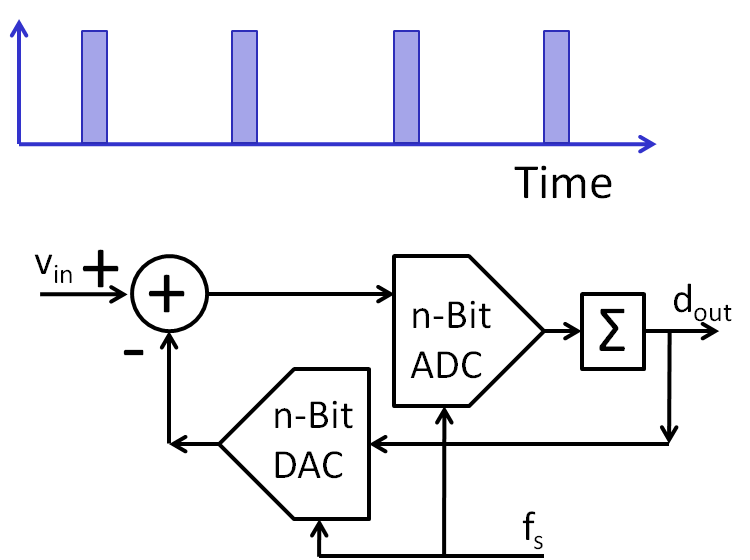1st order passive Sigma Delta Converters 2nd order active Sigma Delta Converters Higher order active Sigma Delta Converters Mashed Sigma Delta Converters Signal to noise ratio Transfer function and stability Voltage range preventing saturation Digital signal processing: filter, decimation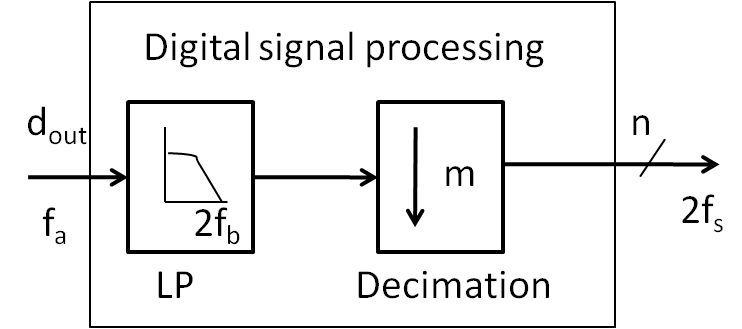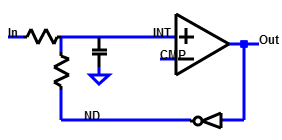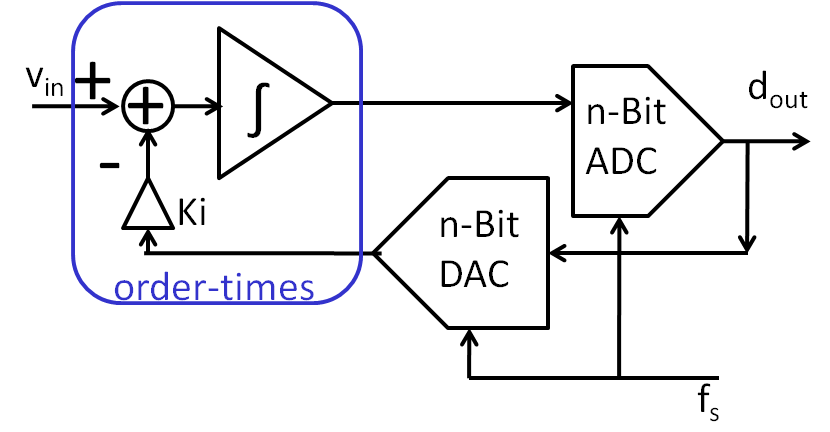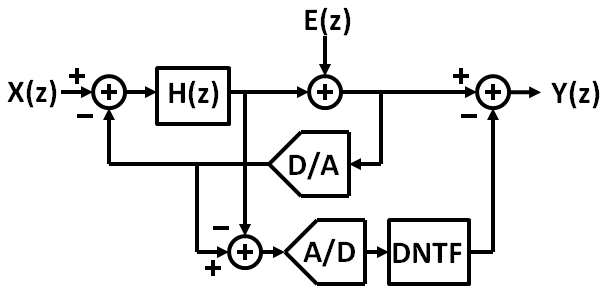Questions

How to build a sigma delta ADC?

• Literature, Theory:
Baker, Razavi, Holberg, Wikipedia, SSCC
• LTSPICE simulation
• Digital filter
• Need for high level simulation
• Realization, Measurement
• Review, Comparison

Overview

• Internal voltages: LTSPICE, high level simulation
• Transfer function: Stability and noise shaping (SNR)
• Signal to noise ratio and architectures
First order, second order, higher order
• Digital filters
Digital time domain circuits and frequency representation
Realization
• Example realization
Passive
Active: continous time realization (CT)
Active: switched capacitor realization (SC)
• CIFB architecture

First order passive Sigma Delta Modulator

Internal voltage levels:

The voltage V(INT n+1) at clock cycle n+1 is:

$V_{int n+1} = V_{int n} + \frac{\delta t}{C} \left( \frac{ V_{not(Dout)} - V_{int n}}{R} + \frac{V_{in} - V_{int n}}{R} \right)$
$V_{int n+1} = V_{int n} + \frac{\delta t}{C \cdot R} \left( V_{not(Dout)} + V_{in} - 2 \cdot V_{int n} \right)$

$\delta t$ is the period of the clock.
$V_{Dout}$ in a real logic circuit is 0V or VDD.

These equations are used for a high level simulation.
High level simulation: 1st order sigma delta simulation

Since Vint has to stay between 0V and VDD:
$\frac{\delta t}{C \cdot R} 2 V_{DD} \lt V_{DD}$
$C \cdot R \gt \frac{2}{f_{sample} }$
$\frac{1}{C \cdot R} \lt \frac{f_{sample} }{2}$
The bandwidth limit of RC has to be smaller than fsample/2.
The bandwidth limit of RC has to be greater than the interested bandwith. fsample/2/OSR
$2 \pi f_{bw} \lt \frac{1}{C \cdot R} \lt \frac{f_{sample} }{2}$
On the left the difference of V(in) and V(D) is integrated and then compared with V(CMP). The comparator operates with the clock frequency and gives for each clock cycle a new value.
A simple passive first order sigma delta converter uses resistors and capacitances.
This circuit is according to Baker, "CMOS integrated cicuits", Figure 6.4

Example: First order passive Sigma Delta Modulator

The circuit with R = 1 kΩ, C= 20 nF is operated with VDD = 1 V, VCMP = 0.5 V and fS = 1 MHz.
What is the maximum allowed input voltage?
What is the cut-off frequency of the RC input low pass?
Calculate vout(t) for 4µs if vin=0.3V. The starting value for vint=VCM and vout=0V. Assume a constant current through R for a sampling cycle.
 t [µs] 0 1 2 3 4 vin 0.3V vint 0.5V vout 0V

1st order passive Sigma Delta high level simulation

High level simulation: 1st order sigma delta simulation

• Internal voltage simulation: Verification of result from previous calculation
• Ramp test: Dead zones and noisy transition
• Digital filter realisation: Averaging, SINC, droop, bitwidth
• FFT: Signal to noise improvement with OSR and filter

General signal and noise transfer function

A first order sigma delta analog digital converter.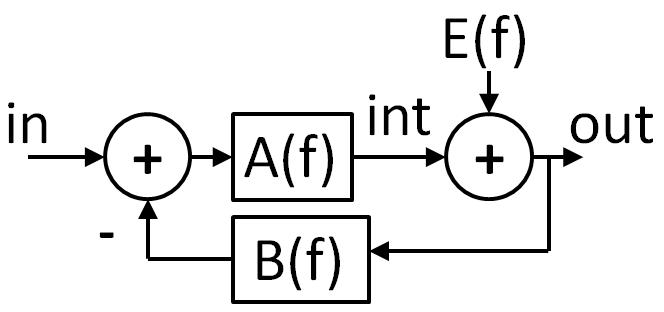$V_{out}(f) = V_{in}(f) \frac{A(f)}{1 + B(f) A(f)} + E(f) \frac{1}{1 + B(f) A(f)}$

$V_{out}(f) = V_{in}(f) \frac{1}{B(f) + \frac{1}{A(f)}} + E(f) \frac{1}{1 + B(f) A(f)}$

There is a signal transfer function and a noise transfer function.

$V_{out}(f) = \left( V_{in}(f) - B(f) V_{out}(f) \right) A(f) + E(f)$
$V_{out}(f) = V_{in}(f) A(f) - B(f) V_{out}(f) A(f) + E(f)$
$V_{out}(f) \left( 1 + B(f) A(f) \right) = V_{in}(f) A(f) + E(f)$
$V_{out}(f) = V_{in}(f) \frac{A(f)}{1 + B(f) A(f)} + E(f) \frac{1}{1 + B(f) A(f)}$
Signal transfer function (STF):
$STF(f) = \frac{A(f)}{1 + B(f) A(f)}$
Noise transfer function (NTF):
$NTF(f) = \frac{1}{1 + B(f) A(f)}$

First order passive Sigma Delta Modulator signal and noise transfer function

$\frac{v_{in} - v_{int}}{R} + \frac{-v_{out} - v_{int}}{R} = i_{int}$
$v_{in} - v_{int} - v_{out} - v_{int} = i_{int} R$
$v_{in} - v_{out} - 2 \cdot v_{int} = i_{int} R$
$v_{in} - v_{out} = i_{int} R + 2 \cdot v_{int}$
$i_{int} = v_{int} j \omega C$
$v_{int} = \frac{v_{in}-v_{out}}{j \omega C R + 2}$
vint = A(f) · (vin - B(f) vout)
$B(f) = 1$      $A(f) = \frac{1}{j \omega C R + 2}$
$V_{out}(f) = \frac{V_{in}}{C R} \frac{1}{j \omega + \frac{3}{R C}} + E(f) \frac{j \omega + \frac{2}{R C}}{j \omega + \frac{3}{R C}}$
Comparing the equation for the internal voltage vint with the general signal and noise transfer function gives:
$V_{int}(f) = A(f) \cdot ( V_{in} - V_{out} \cdot B(f) )$
$B(f) = 1$
$A(f) = \frac{1}{j \omega C R + 2}$
$V_{out}(f) = V_{in} \frac{1}{j \omega C R + 3} + E(f) \frac{j \omega C R + 2}{j \omega C R + 3}$
$V_{out}(f) = \frac{V_{in}}{C R} \frac{1}{j \omega + \frac{3}{R C}} + E(f) \frac{j \omega + \frac{2}{R C}}{j \omega + \frac{3}{R C}}$

Noise shaping

 Signal pole: ω=3/(RC) Noise zero: ω=2/(RC) Noise pole: ω=3/(RC) Position fclk Position fbw = 3/(2 π R C) Noise is digitally filtered. $\epsilon_q = \frac{LSB}{\sqrt{12}}$ $- 20 log \sqrt{12} = -10.8 dB$

Digital filter and decimator

 SINC Filter Filter order: SINC, SINC2, SINC3 The order of the filter has to be one more than the sigma delta modulator to realize the full signal to noise benefit.A decimating Sinc2 filter has the following schematic with 2 integrators and 2 differentiators: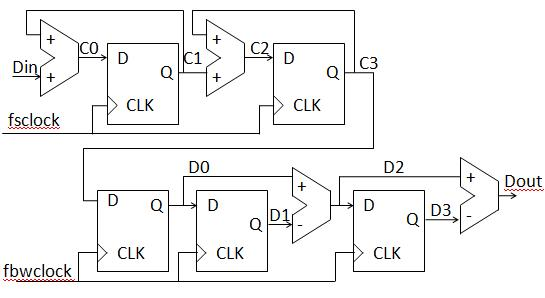How to physically realize the filter?
Width of the registers?

Calculating Signal to noise ratio

$V_{noise,RMS}^{2} = 2 \int_0^{BW}{|NTF(f)|^2|V_{Qe}(f)|^2} df$
Quantization noise spectral density divides the quantization error with sampling frequency:
$|V_{Qe}(f)|^2 = \frac{V_{LSB}^{2}}{12 f_{sample}}$
Transfer function:
$V_{out}(f) = STF(f) v_{in} + NTF(f) V_{Qe}$
First order passive modulator :
$V_{out}(f) = \frac{V_{in}}{C R} \frac{1}{j \omega + \frac{3}{R C}} + \frac{E(f)}{R C} \frac{j \omega R C + 2}{j \omega + \frac{3}{R C}}$
Since signal transfer function and noise transfer function have the part:
$STF(f) = \frac{1}{j \omega + \frac{3}{RC}}$
This cancels out for signal to noise ratio.

Calculating Signal to noise ratio (continued)

$V_{noise,RMS}^{2} = 2 \frac{V_{LSB}^{2}}{12 f_{sample}} \int_0^{BW}{(2 \pi f R C + 2)^2} df$
$V_{noise,RMS}^{2} = 2 \frac{V_{LSB}^{2}}{12 f_{sample}} \left( {(2 \pi R C )^2} \frac{BW^3}{3} + 4 \pi R C \frac{BW^2}{2} + 4 BW \right)$
Sinc-shaped lowpass filter:
$BW = \frac{f_{sample}}{2 OSR}$

First part only: $V_{noise,RMS}^{2} = \frac{V_{LSB}^{2}}{12 } {(2 \pi R C)^2} \frac{f_{sample}^2}{12 OSR^{3}}$

$SNR = 20 log\frac{V_{signal,rms}}{V_{noise, rms}}$
$SNR = 6.02 N + 1.76 - 20 log \frac{(2 \pi R C) f_{sample}}{\sqrt{12}} + 20 log OSR^{\frac{3}{2}}$

$SNR = 6.02 N + 1.76 - 20 log \frac{(2 \pi R C) f_{sample}}{\sqrt{12}} + 30 log OSR$
With no oversampling signal to noise ratio decreases by:
$d SNR = - 20 log \frac{(2 \pi R C ) f_{sample}}{\sqrt{12}}$
The maximum value for fRC = 1 / (2 π R C) = 0.5 f sample
. $d SNR = -20 log(\frac{2}{\sqrt{12}}) = 4.77 dB$ With larger oversampling rates signal to noise ratio improves by 30 log 2 = 9 dB, 9 dB / 6 dB = 1.5 bits for doubling OSR.

Increasing signal to noise with oversampling

 Quantization error: $SNR = 6.02 B dB + 1.76 dB$ Averaging: $SNR = 6.02 B dB + 1.76 dB + 10 \cdot log( OSR )$ Increased Resolution: $B = \frac{10 \cdot log( OSR )}{6.02}$ 1st order sigma delta $SNR = 6.02 B dB + 1.76 dB - 5.17 dB + 30 \cdot log( OSR )$ Increased Resolution: $B = \frac{30 \cdot log( OSR ) - 5.17 }{6.02}$ 2nd order sigma delta $SNR = 6.02 B dB + 1.76 dB - 12.9 dB + 50 \cdot log( OSR )$ Increased Resolution: $B = \frac{50 \cdot log( OSR ) - 12.9 }{6.02}$ General limit $SNR_{max} = 10 \cdot log\left( \frac{3(2n+1)}{2 \pi^{2n}} OSR^{2n+1}\right) dB$ $SNR_{max} = 10 \cdot log\left( \frac{3(2n+1)}{2 \pi^{2n}}\right) + 10 \cdot (2 \cdot n + 1) \cdot log\left( OSR\right) dB$ OSR: oversampling rate n: order of sigma delta modulator

Graph increasing signal to noise with oversampling

 Example: fbw = 1 kHz 1. Order 1-bit sigma delta 8-bit resolution desired.

Signal to noise, FFT, transfer characteristic and oversampling

• Quantization noise is spread over the number of FFT bins NFFT:
$\Delta SNR = 10 log\frac{N_{FFT}}{2}$
• Averaging can improve signal to noise ratio
FFT: Half number of points, oversampling with ratio of OSR = 2, reduction of noise
$\Delta SNR = 10 log OSR$
Increase of number of bits: OSR = 2 gives Δ SNR = 3 dB is 1/2 bit
Example: 8-bit signal with 4-bit noise amplitude.
Signal: $V_{rms} = \frac{V_{amplitude}}{\sqrt{2}}$
Noise: Vrms = VPP/6 = Vamplitude/3

 Averaging (OSR) Bits Max value ENOB 1 8 255 5 4 10 1023 6 16 12 4095 7 256 16 65355 9

Reference: CMOS Analog Circuit Design, Allen, Holberg

Chapter 10.9: Oversampling Converters

•  Single loop 7th order 118 dB (19 dB)
•  Single loop 5th order 20 bit:
Thomsen, Bemades, "A digitally Corrrected 20-bit Delta Sigma Modulator", ISSCC, 194-195, Feb 1994
• [60-64] 3rd order: 2-1 MASH
Longo, Copeland, "A 13-bit ISDN-Band Oversampled ADC..", CICC, pp.21.2.1-21.2.4, Jan 1988
Williams, Wooley, "A Third order Sigma-Delta..", JSSC, Vol 29. No. 3, pp.193-202,Mar 1994
Yin, Stubbe, Sansen, "Av16-bit 320 kHz CMOS ADC..", JSSC, Vol. 28, No.6, pp.640-647, June 1993
Rabii, Wooley, " A 1.8-V 0.8um CMOS ADC", JSSC, Vol 32., No. 6, pp.783-796, June 1993
Brandt, Wooley, "50MHz 12b 2MHz ADC", JSSC,Vol. 26, No. 6, pp. 1746-1756, Dec 1991
• [65-67] 4th order: 2-2 MASH
Tenhunen, "An oversampled ..", ISCAS, pp. 3279-3282, May 1990
Ritoniemi et. al., "A Stereo Audio..", JSSC, Vol. 29, No. 12, pp. 1514-1523, Dec 1994
Fujimori et al., "A 5-V 111dB dynamic range", JSSC, Vol. 32, No. 3, pp.329-336, Mar 1997
•  6th order: 2-2-2 MASH
Dedic, " A sixth order", ISSCC Dig. Tech. Papers, pp. 188-189, Feb 1994
•  MASH Decimator: Candy,Temes, oversampling Delta-Sigma Data Converters. IEEE Press
• INF4420 Projects in analog/mixed signal CMOS design

Practical realization of a 1st order Sigma Delta converter

Real 1st order passive sigma delta modulator

Hardware: Digilent NEXYS 3 board with Xilinx FPGA tCLK = 100MHz.

Objectives and Challenges

Generate high resolution sine signal
Analysis of different R, C components, oversampling and timing
Data transfer and processing